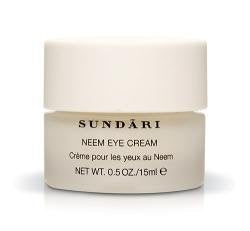# Neem Eye Cream - 0.5 Oz.

• \$66.48
• Save \$28.23

• N

• e

• e

• m

• E

• y

• e

• c

• r

• e

• a

• m

• i

• s

• h

• e

• a

• l

• i

• n

• g

• a

• n

• d

• r

• e

• d

• u

• c

• e

• s

• p

• u

• f

• f

• i

• n

• e

• s

• s

• f

• r

• o

• m

• a

• r

• o

• u

• n

• d

• t

• h

• e

• e

• y

• e

• a

• r

• e

• a

• .

Sundari Neem Eye Cream -- 0.5 oz.

Neem Eye Cream is healing and reduces puffiness and dark circles from around the eye area.

every pages